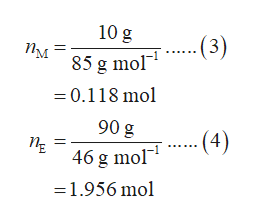# A solution of methylene chloride (CH2Cl2) and ethanol (CH3CH2OH) that is 10.% methylene chloride by mass is boiling at 75°C. The vapor is collected and cooled until it condenses to form a new solution.Calculate the percent by mass of methylene chloride in the new solution. Here's some data you may need: normal boiling pointdensityvapor pressure at 75°Cmethylene chloride40.°C1.3g/mL2263.torrethanol78.°C0.79g/mL669.torrBe sure your answer has 2 significant digits. Note for advanced students: you may assume the solution and vapor above it are ideal.

Question
2 views

A solution of methylene chloride (CH2Cl2) and ethanol (CH3CH2OH) that is 10.% methylene chloride by mass is boiling at 75°C. The vapor is collected and cooled until it condenses to form a new solution.

Calculate the percent by mass of methylene chloride in the new solution. Here's some data you may need:

 normal boiling point density vapor pressure at  75°C methylene chloride 40.°C 1.3g/mL 2263.torr ethanol 78.°C 0.79g/mL 669.torr

Note for advanced students: you may assume the solution and vapor above it are ideal.

check_circle

Step 1

The mole fraction of methyl chloride (χM) at 750C is calculated as shown in equation (1) where P0M is the vapor pressure of methyl chloride, P0E is the vapor pressure of ethanol, and PT is the total vapor pressure.

Step 2

Consider the total mass (wT) of the solution is 100 g. The mass of methyl chloride is calculated as shown in equation (2) where w/w% is equal to 10% of methyl chloride and wM is the mass of methyl chloride. Thus, in 100 g of solution, the mass of methyl chloride is 10 g and the mass of ethanol is 90 g.

Step 3

The number of moles of methyl chloride (n­M) in 10 g of methyl chloride and the number of moles ethanol (nE) in 90 g ethanol is calculated as shown in equation (3) and (4) respectively where MM and ME are the molar m...help_outlineImage Transcriptionclose10 g ..(3) Пм 85 g mol 0.118 mol 90 g - (4) 46 g mol =1.956 mol fullscreen

### Want to see the full answer?

See Solution

#### Want to see this answer and more?

Solutions are written by subject experts who are available 24/7. Questions are typically answered within 1 hour.*

See Solution
*Response times may vary by subject and question.
Tagged in

### Chemistry